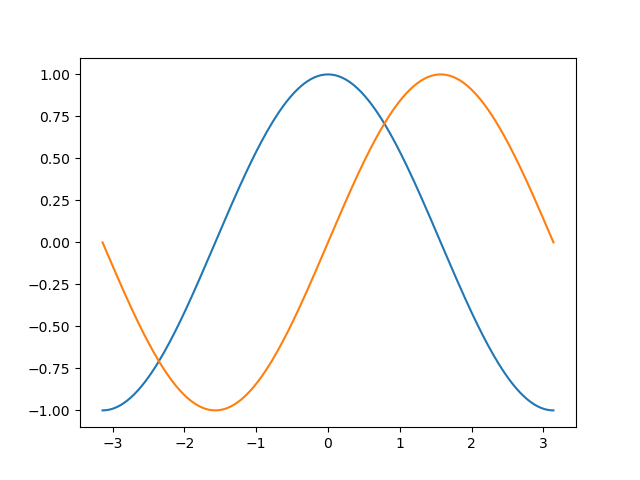# Excercise 1¶

Solution of the excercise 1 with matplotlib.```import numpy as np
import matplotlib.pyplot as plt

n = 256
X = np.linspace(-np.pi, np.pi, 256)
C,S = np.cos(X), np.sin(X)
plt.plot(X, C)
plt.plot(X,S)

plt.show()
```

Total running time of the script: ( 0 minutes 0.017 seconds)

Gallery generated by Sphinx-Gallery